Select Page

# Linear equations

Linear equations are expressions that can reduce to the form ax+b=0, with a≠0. Their only solution is x=-(b/a) and the unknown x only appears raised to 1.

Examples linear equations: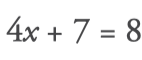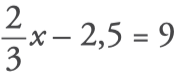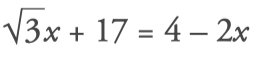Examples of nonlinear equations: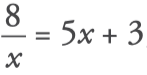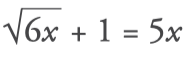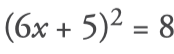## Equivalent equations

Two equations are equivalent if they have the same solution or both have no solution. Thus, the equations 5x–9=51 and 3x-7=89–5x are equivalent because the solution of both is x=12.
To solve an equation, we have to clear the x using a series of steps. Each step consists of transforming the equation into another equivalent equation, in which the x is closest to being cleared.

## How to solve linear equations

Keep in mind the following rules to avoid making mistakes:

• Add or subtract the same expression in the two members of the equality. That is, what is adding in one member passes by subtracting the other member, and vice versa.
• Multiply or divide the two members by the same non-zero number. In other words, what is multiplying everything else from one member happens dividing the other, and vice versa.

How to solve a linear equation:

1. Remove denominators, if any.

For this, the two members of the equation are multiplied by a common multiple of the denominators; preferably, their least common multiple: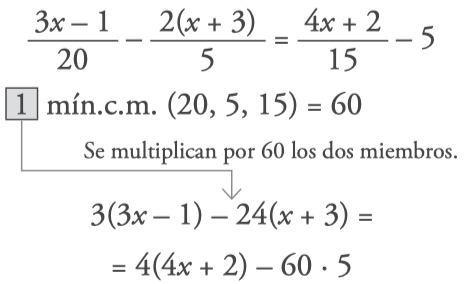2. Remove parentheses, if any.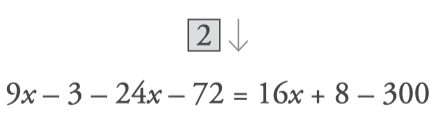3. Pass the x terms to one member and the numbers to the other member: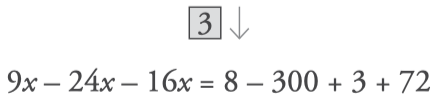4. Simplify each member: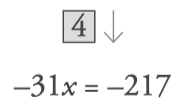5. Clear the x and get the solution like this: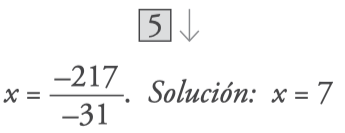6. Check by substituting the x for the solution obtained.

Since they match, the solution is correct: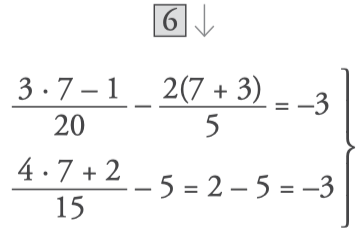The best way to learn to solve linear equations is to practice doing lots of exercises. Start now and test yourself!

## Linear equation exercises

1. Find out the value of x:

## Ejercicios de ecuaciones

2. Solve the following equations:

## Ejercicios de ecuaciones

### Factors of 36

The number 36 has 9 factors and is a composite number. To calculate the factors of 36 we divide,...

### Factors of 35

The number 35 has 4 factors and is a composite number. To calculate the factors of 35 we divide,...

### Factors of 34

The number 4 has 4 factors and is a composite number. To calculate the factors of 34 we divide,...

### Factors of 33

The number 33 has 4 factors and is a composiite number. To calculate the factors of 33 we divide,...

### Factors of 32

The number 32 has 6 factors and is a composite number. To calculate the factors of 32 we divide,...

### Factors of 31

The number 31 has 2 factors and is a prime number. To calculate the factors of 31 we divide,...

### Factors of 30

The number 30 has 8 factors and is a composite number. To calculate the factors of 30 we divide,...

### Factors of 29

The number 29 has 2 factors and is a prime number. To calculate the factors of 29 we divide,...

### Factors of 28

The number 28 has 5 factors and is a composite number. To calculate the factors of 28 we divide,...

### Factors of 27

The number 27 has 4 factors and is a composite number. To calculate the factors of 27 we divide,...